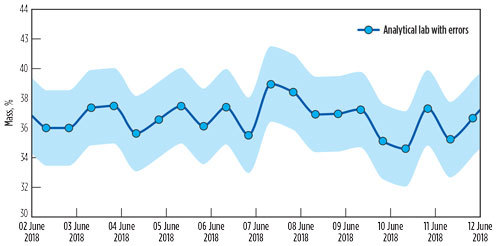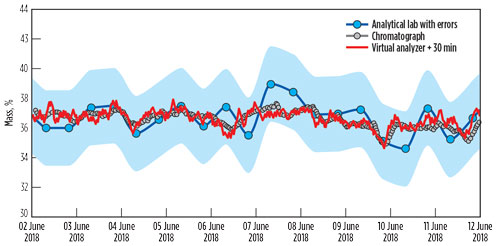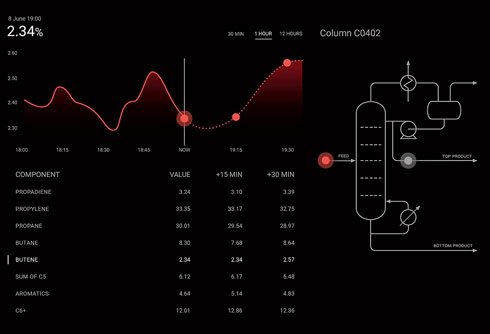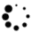# Implement AI to predict the composition of chemical plant feed

An important indicator of the technological processes at chemical plants is the composition of the main flows, including feed, products and intermediate flows.

An important indicator of the technological processes at chemical plants is the composition of the main flows, including feed, products and intermediate flows. It is believed that, at present, the accuracy of determining the composition by modern methods of analysis is quite high, but the error in determining the concentration of components may exceed permissible values for many petrochemical processes. At the same time, analysis of the composition of process flows is not usually provided for all potentially important flows, due to relatively high investment and operating costs.

The use of the latest developments in the technologies of artificial intelligence (AI) and machine learning has great potential to reduce the cost of work to determine the composition of process flows while increasing the quality of the results.

## Existing methods for determining process stream composition

A common method for determining the concentration of components in process streams is inline chromatography analysis. Analysis is carried out during a specified cycle of the analyzer, which can span 30 min–60 min.

Generally, the accuracy of determining the composition by means of such an analyzer is not adequately high. This is due to a decrease in sensitivity to the concentrations of components, which happens due to the high frequency of measurements—one analysis per 30 min–60 min. In due course, each chromatographic analyzer must be disconnected from the industrial plant and calibrated.

The main tool and resource for determining the most accurate composition of the process flow is an analytical laboratory. As a rule, the frequency of analysis ranges from 12 hr–24 hr. The sampling and analysis procedures, including fixing the exact time of sampling, must be regulated so that the results of laboratory analyses have an applied value in the condition of varying process flows.

Despite a more precise determination of the composition of the samples, the analytical laboratory cannot always be considered as a “gold standard,” because the results may have some systematic error—or, in other words, a confidence interval, which is the average value of the error of the results of the analytical laboratory. The authors encountered such an error while carrying out a full-scale experiment on the feed flow at a depropanizer column. They took several samples in a row from a process flow with a short time interval and at a stable process regime. The analysis results were not identical, but they were within the confidence interval characterized by a certain systematic error.

## Full-scale experiment

To determine the confidence interval of the analytical laboratory, a test was carried out at the depropanizer column for the separation of C3 hydrocarbons. The feed pipeline was sampled within 2 hr in a row (from 12:00 to 14:00), with an interval of 5 min. The content of components in the analytical laboratory were subsequently analyzed. The tests results are shown in Table 1.

According to the presented data, between 5-min measurements, the results of the analysis of the analytical laboratory for each component, on average, have the following variations:

• Propadiene = 0.88 wt%, which is 22.4% of the average component concentration
• Propylene= 1.85 wt%, which is 5.5% of the average component concentration
• Propane = 2.32 wt%, which is 7.5% of the average component concentration
• n-Butane + i-Butane = 0.86 wt%, which is 10.8% of the average component concentration
• Butene = 0.95 wt%, which is 30.4% of the average component concentration
• Sum of C5 = 1.4 wt%, which is 21.7% of the average component concentration
• Aromatics = 1.1 wt%, which is 20.2% of the average component concentration
• С6+ = 3.7 wt%, which is 47.6% of the average component concentration.

According to the results of the analysis of components in the process flow, at a 5-min sampling period, a high-value scattering is noticeable. For this case, the values of the confidence intervals are presented in Table 2.

Fig. 1 shows the values of the analytical laboratory results for propane, along with the confidence interval from Table 2.

## Implementing AI to determine feed flow composition

Alongside the results of chromatographic analyses, petrochemical and oil refineries measure and record other technological parameters. These data are used to monitor and control the process blocks and the plant as a whole. The volume of these measurements is large and, at present, this data contains the potential for additional optimization of units without the use of hardware or constructive intervention in the plant.FIG. 1. Values of the analytical laboratory results for the propane, along with the confidence interval.

By using statistical methods of data analysis based on AI and machine learning, it is possible to determine parameters that are not measured directly or indirectly by the sensors of a depropanizer column. The calculation is based on thermodynamic and statistical dependencies, which could be found in changing the parameters of the plant. This allows for the calculation of additional process parameters, based on the values of the process sensors. At the same time, to calculate such new parameters, physical access to the process flows is not required. Physical access is necessary only to organize access to the database, which contains the actual values of the parameters of the chemical process.

In addition to determining parameters that are not measured, it is possible to create a digital analog of an existing chromatographic analyzer. At the same time, it is possible to add new functions—for example, the prediction of the concentration of components in the process flow for some limited future horizon (i.e., predicting changes in composition).FIG. 2. Results of the calculation of the mathematical model were within the confidence interval of the analytical laboratory.

For the depropanizer column, a mathematical model and software based on AI technologies were developed to calculate the concentration of components in the feed flow of the unit.

As shown in Fig. 2, the results of the calculation of the mathematical model—which was applied to the calculation of the composition of the feed of the unit without physical access to the process flow—were within the confidence interval of the analytical laboratory.

At the same time, mathematical models for the prediction of the composition of the depropanizer column feed flow in a future horizon of 30 min were developed. Fig. 3 shows the predicted values of the concentration of the propane received with the help of such a mathematical model. The values do not exceed the limits of the confidence interval of the analytical laboratory.FIG. 3. Predicted values of the concentration of the propane received with the help of a mathematical model.

The accuracy of parameter value calculation by mathematical models could be compared to the accuracy of parameter value determination by physical measuring instruments. As such, the replacement of expensive in-service sensors that use digital analogs based on mathematical models can be considered.

The mathematical model can be located on any digital medium with an operating system, screen and access to the database of the petrochemical plant. This greatly simplifies the process and the cost of integration, since it does not require any changes to the internal information structure of the chemical manufacture.

For the depropanizer column, mathematical models were installed on a portable tablet computer with a pre-installed set of algorithms and the possibility of installation of mathematical models. Calculation of the additional parameters of the chemical plant in the automatic mode was achieved on the basis of the measured values of technological parameters.FIG. 4. Interface displaying calculation results, a table of values of the components concentration and a schematic diagram of the unit.

Connection to the depropanizer column database was made via USB protocol. Mathematical models were used to calculate the values of technological parameters in real time. The values were calculated for the current point of time and for 15 min and 30 min in advance. The calculation results are displayed on the interface (Fig. 4), which contains a graphic display area, a table of values of the components concentration and a schematic diagram of the unit. Indicated flows for which the calculation of values was performed are on the schematic diagram. Integration took one working day and did not require additional financial costs.

## Takeaway

This example can be scaled for other petrochemical processes and for other industries. It is possible to simultaneously implement such analyzers for different process flows of a plant, allowing the manufacturer to receive a complete picture of operations and improve the efficiency of plant management. HP

## From the Archive